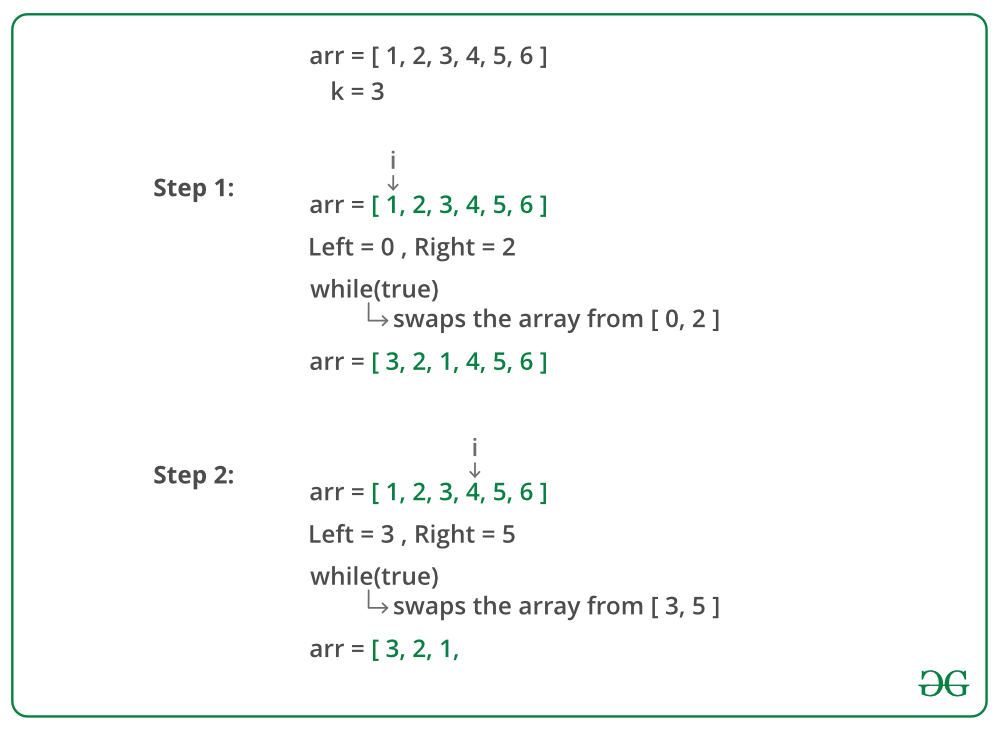# Reverse an array in groups of given size

• Difficulty Level : Easy
• Last Updated : 25 Jun, 2021

Given an array, reverse every sub-array formed by consecutive k elements.

Examples:

Input:
arr = [1, 2, 3, 4, 5, 6, 7, 8, 9]
k = 3
Output:
[3, 2, 1, 6, 5, 4, 9, 8, 7]

Input:
arr = [1, 2, 3, 4, 5, 6, 7, 8]
k = 5
Output:
[5, 4, 3, 2, 1, 8, 7, 6]

Input:
arr = [1, 2, 3, 4, 5, 6]
k = 1
Output:
[1, 2, 3, 4, 5, 6]

Input:
arr = [1, 2, 3, 4, 5, 6, 7, 8]
k = 10
Output:
[8, 7, 6, 5, 4, 3, 2, 1]

Approach: Consider every sub-array of size k starting from the beginning of the array and reverse it. We need to handle some special cases. If k is not multiple of n where n is the size of the array, for the last group we will have less than k elements left, we need to reverse all remaining elements. If k = 1, the array should remain unchanged. If k >= n, we reverse all elements present in the array.

Below image is a dry run of the above approach:Below is the implementation of the above approach:

## C++

 `// C++ program to reverse every sub-array formed by``// consecutive k elements``#include ``using` `namespace` `std;` `// Function to reverse every sub-array formed by``// consecutive k elements``void` `reverse(``int` `arr[], ``int` `n, ``int` `k)``{``    ``for` `(``int` `i = 0; i < n; i += k)``    ``{``        ``int` `left = i;` `        ``// to handle case when k is not multiple of n``        ``int` `right = min(i + k - 1, n - 1);` `        ``// reverse the sub-array [left, right]``        ``while` `(left < right)``            ``swap(arr[left++], arr[right--]);` `    ``}``}` `// Driver code``int` `main()``{``    ``int` `arr[] = {1, 2, 3, 4, 5, 6, 7, 8};``    ``int` `k = 3;` `    ``int` `n = ``sizeof``(arr) / ``sizeof``(arr);` `    ``reverse(arr, n, k);` `    ``for` `(``int` `i = 0; i < n; i++)``        ``cout << arr[i] << ``" "``;` `    ``return` `0;``}`

## Java

 `// Java program to reverse every sub-array formed by``// consecutive k elements``class` `GFG {``    ` `    ``// Function to reverse every sub-array formed by``    ``// consecutive k elements``    ``static` `void` `reverse(``int` `arr[], ``int` `n, ``int` `k)``    ``{``        ``for` `(``int` `i = ``0``; i < n; i += k)``        ``{``            ``int` `left = i;``    ` `            ``// to handle case when k is not multiple``            ``// of n``            ``int` `right = Math.min(i + k - ``1``, n - ``1``);``            ``int` `temp;``            ` `            ``// reverse the sub-array [left, right]``            ``while` `(left < right)``            ``{``                ``temp=arr[left];``                ``arr[left]=arr[right];``                ``arr[right]=temp;``                ``left+=``1``;``                ``right-=``1``;``            ``}``        ``}``    ``}``    ` `    ``// Driver method``    ``public` `static` `void` `main(String[] args)``    ``{``        ` `        ``int` `arr[] = {``1``, ``2``, ``3``, ``4``, ``5``, ``6``, ``7``, ``8``};``        ``int` `k = ``3``;``    ` `        ``int` `n = arr.length;``    ` `        ``reverse(arr, n, k);``    ` `        ``for` `(``int` `i = ``0``; i < n; i++)``            ``System.out.print(arr[i] + ``" "``);``    ``}``}` `// This code is contributed by Anant Agarwal.`

## Python3

 `# Python 3 program to reverse every``# sub-array formed by consecutive k``# elements` `# Function to reverse every sub-array``# formed by consecutive k elements``def` `reverse(arr, n, k):``    ``i ``=` `0``    ` `    ``while``(i

## C#

 `// C# program to reverse every sub-array``// formed by consecutive k elements``using` `System;` `class` `GFG``{` `// Function to reverse every sub-array``// formed by consecutive k elements``public` `static` `void` `reverse(``int``[] arr,``                           ``int` `n, ``int` `k)``{``    ``for` `(``int` `i = 0; i < n; i += k)``    ``{``        ``int` `left = i;` `        ``// to handle case when k is``        ``// not multiple of n``        ``int` `right = Math.Min(i + k - 1, n - 1);``        ``int` `temp;` `        ``// reverse the sub-array [left, right]``        ``while` `(left < right)``        ``{``            ``temp = arr[left];``            ``arr[left] = arr[right];``            ``arr[right] = temp;``            ``left += 1;``            ``right -= 1;``        ``}``    ``}``}` `// Driver Code``public` `static` `void` `Main(``string``[] args)``{``    ``int``[] arr = ``new` `int``[] {1, 2, 3, 4,``                           ``5, 6, 7, 8};``    ``int` `k = 3;` `    ``int` `n = arr.Length;` `    ``reverse(arr, n, k);` `    ``for` `(``int` `i = 0; i < n; i++)``    ``{``        ``Console.Write(arr[i] + ``" "``);``    ``}``}``}` `// This code is contributed``// by Shrikant13`

## PHP

 ``

## Javascript

 ``

Output:

`3 2 1 6 5 4 8 7`

Time complexity of above solution is O(n).
Auxiliary space used by the program is O(1).